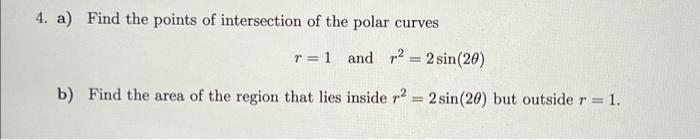# Question 4. a) Find the points of intersection of the polar curves $r=1 \text { and } r^{2}=2 \sin (2 \theta)$ b) Find the area of the region that lies inside $$r^{2}=2 \sin (2 \theta)$$ but outside $$r=1$$.Transcribed Image Text: 4. a) Find the points of intersection of the polar curves $r=1 \text { and } r^{2}=2 \sin (2 \theta)$ b) Find the area of the region that lies inside $$r^{2}=2 \sin (2 \theta)$$ but outside $$r=1$$.
Transcribed Image Text: 4. a) Find the points of intersection of the polar curves $r=1 \text { and } r^{2}=2 \sin (2 \theta)$ b) Find the area of the region that lies inside $$r^{2}=2 \sin (2 \theta)$$ but outside $$r=1$$.&#12304;General guidance&#12305;The answer provided below has been developed in a clear step by step manner.Step1/2a)Given polar curves are$$\mathrm{{r}={1}}$$$$\mathrm{{r}^{{2}}={2}{\sin{{\left({2}\theta\right)}}}}$$To find the points of intersectionsubstitute one equation with another equationthen we get $$\mathrm{{1}={2}{\sin{{\left({2}\theta\right)}}}}$$$$\mathrm{{\sin{{\left({2}\theta\right)}}}=\frac{{1}}{{2}}}$$$$\mathrm{{\sin{{\left({2}\theta\right)}}}={\sin{{\left({2}{n}\pi+{30}^{{o}}\right)}}}}$$where $$\mathrm{{n}={0},{1},{2},{3},{4}\ldots.}$$then $$\mathrm{{2}\theta={2}{n}\pi+{30}^{{o}}}$$$$\mathrm{\theta={n}\pi+{15}^{{o}}}$$ where $$\mathrm{{n}={0},{1},{2},{3},{4},\ldots.}$$Explanation:Please refer to solution in this step.Step2/2b)To find the area of the region that lies inside $$\mathrm{{r}^{{2}}={2}{\sin{{\left({2}\theta\right)}}}}$$but outside $$\mathrm{{r}={1}}$$then in 1st quadrantthe curves meet at pi/8 thenA_between\( \ ... See the full answer